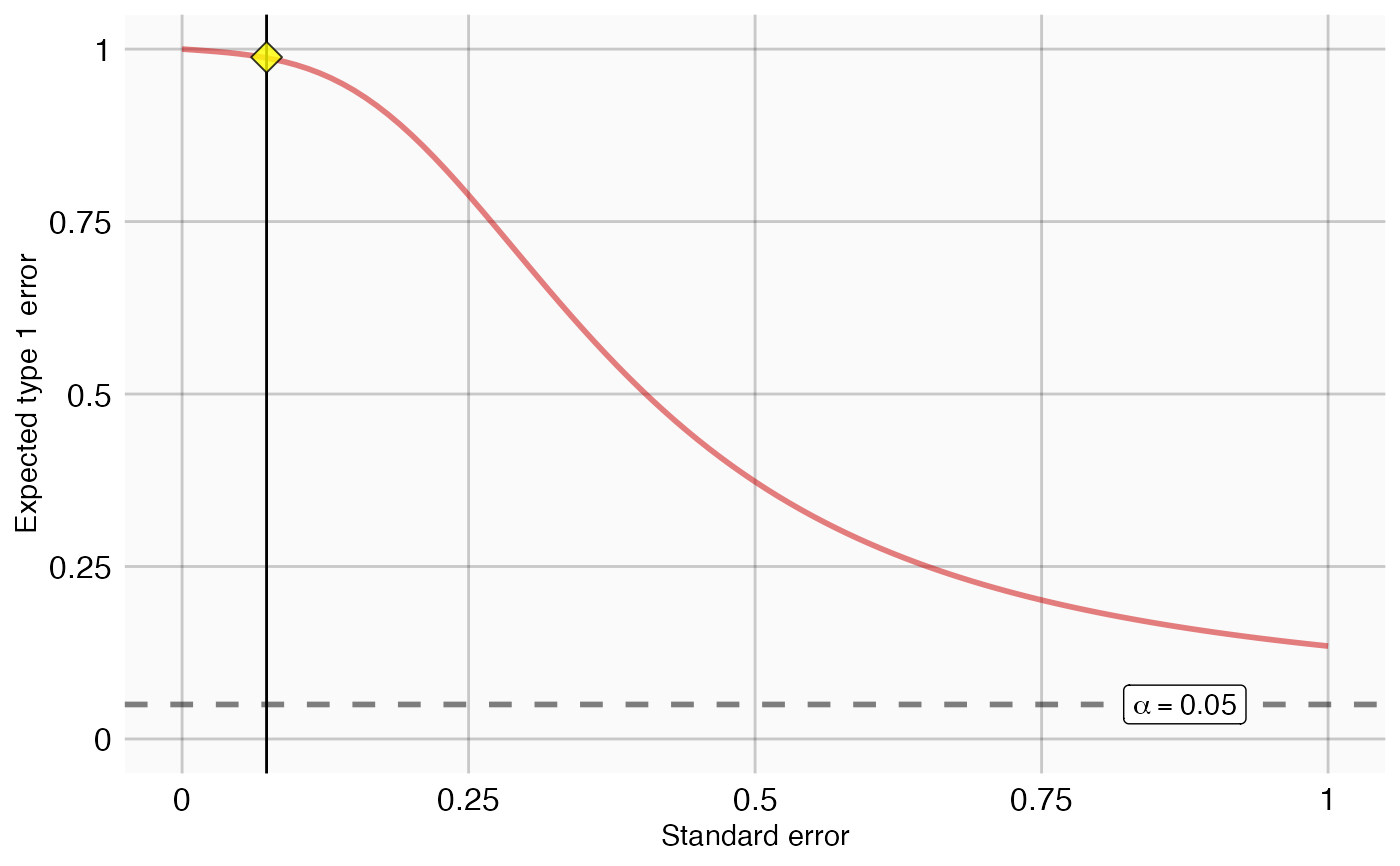plotExpectedType1Error creates a plot showing the expected type 1 error as a function of standard error.

plotExpectedType1Error(
logRrNegatives,
seLogRrNegatives,
seLogRrPositives,
alpha = 0.05,
null = NULL,
xLabel = "Relative risk",
title,
showCis = FALSE,
showEffectSizes = FALSE,
fileName = NULL
)

## Arguments

logRrNegatives

A numeric vector of effect estimates of the negative controls on the log scale.

seLogRrNegatives

The standard error of the log of the effect estimates of the negative controls.

seLogRrPositives

The standard error of the log of the effect estimates of the positive controls.

alpha

The alpha (nominal type 1 error) to be used.

null

An object representing the fitted null distribution as created by the fitNull function. If not provided, a null will be fitted before plotting.

xLabel

If showing effect sizes, what label should be used for the effect size axis?

title

Optional: the main title for the plot

showCis

Show 95 percent credible intervals for the expected type 1 error.

showEffectSizes

Show the expected effect sizes alongside the expected type 1 error?

fileName

Name of the file where the plot should be saved, for example 'plot.png'. See the function ggsave in the ggplot2 package for supported file formats.

## Value

A Ggplot object. Use the ggsave function to save to file.

## Details

Creates a plot with the standard error on the x-axis and the expected type 1 error on the y-axis. The red line indicates the expected type 1 error given the estimated empirical null distribution if no calibration is performed. The dashed line indicated the nominal expected type 1 error rate, assuming the theoretical null distribution.

If standard errors are provided for non-negative estimates these will be plotted on the red line as yellow diamonds.

## Examples

data(sccs)
negatives <- sccs[sccs$groundTruth == 0, ] positive <- sccs[sccs$groundTruth == 1, ]
plotExpectedType1Error(negatives$logRr, negatives$seLogRr, positive\$seLogRr)